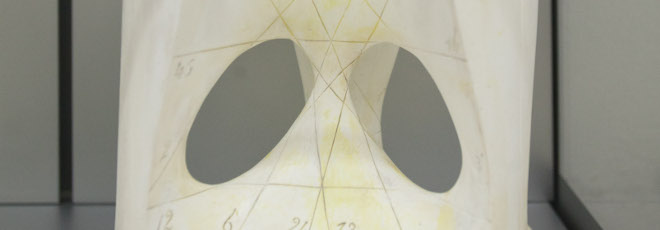## “The Graph p-Laplacian Eigenvalue Problem”

Wednesday 13 April 2022 h. 14:30 - Room 2BC30 and Zoom - Piero Deidda (Padova, Dip. Mat.)

Abstract

In the last years graphs have been used to model a large variety of phenomena, such as the classical transportation networks, the social network interactions, the chemical reactions, ecological interactions. In the study of the structure of a graph, two classical problems are the shortest path problem and the optimal partition or cut problem. Interestingly both of these problems can be related to the p-Laplacian eigenvalue problem and in particular with the two degenerate cases of p=1 and p=infinity. The p-Laplacian eigenvalue problem historically arose from the study of the classical Poincare’ constant and can be addressed as the study of the critical points of the so called “Rayleigh quotient”. It is one of the most classical example of non-linear eigenvalue problem and has been widely studied both in the continuous and discrete setting. We provide a short summary of the main results in this field, highlighting the differences from the linear (p=2) case and the topological information provided by the limit cases p=1 and p=infinity. We will show at the end some original numerical schemes for the computation of the p-eigenpairs.

The video of the seminar will appear shortly afterwards in this Mediaspace channel.

Seminario Dottorato